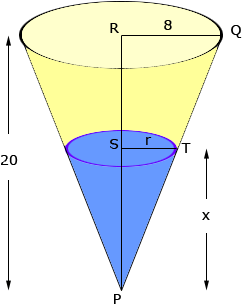Dear Sir, My son has asked me this question but I am somewhat lost. Can you please guide? ------------------------------ A vessel has the shape of an inverted cone.The radius of the top is 8 cm and the height is 20 cm. Water is poured in to a height of x cm.Show that if the volume of the water is V cubic cm,then V=(4/75)pi x3. Thanks & regards, Ghulam Ghulam, The essential concept here is similar triangles.In the diagram above I have let r be the radius of the circle formed by the water surface. The triangles PQR and PTS are similar and hence  |QR|/|RP| = |TS|/|SP|  that is  8/20 = r/x  Hence r = 2/5  x The volume of a cone of radius r and height h is V = 1/3r2 h and hence the volume of water in your cone is V = 1/3r2 x =  1/3( 2/5  x)2 x =  4/75x3 Penny